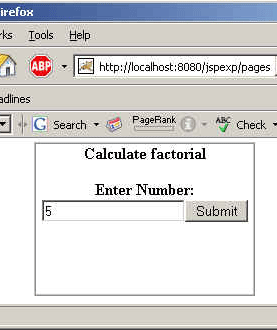# Calculate factorial Using Recursion

After going through this example you will be understand how you can calculate the factorial by using recursion in jsp.# Calculate factorial Using Recursion

After going through this example you will be understand how you can calculate the factorial by using recursion in jsp. To make a program on factorial, firstly it must be clear what is recursion. In a simple language we can define recursion as a programming technique in which a function may call itself. The key to solving recursively is to imagine that your method does what it says.

To make a program on factorial by using recursion in jsp we are going to design a html page named calFactorial.html which will take the number of which you want to calculate the factorial.

The number we have entered in the html page will be retrieved in jsp page by using the method getParameter() method of request  implicit object. Only after that the value of the number can be calculated. Now define the method named factorial with return type of int, it will take one input whose factorial has to be calculated. We have defined this method inside the declaration directive. In the  method we have declared that if the input is 1 then 1 will be returned as a factorial. If the input number is more than 1 then the factorial will be calculated by using recursion.  The output will be displayed to you by using the out implicit object of jsp, out object is responsible for writing the content on the jsp page.

In this program we have used following directives and implicit objects.

Scriptlet: The main logic of the program has to be written here.

Declaration: This is used for declaring variables and methods. Whatever we define in a declaration directive will be applicable in the whole page.

req: It is one of the frequenly used implicit object. It means that this object is applicable until the request object is present.

out: This implicit object is used to write the content on the browser.

The code of the html page is given below.

```<html>
<title>calculate factorial</title>
<body><p>&nbsp;</p>
cellspacing="0" width="35%" align="center">

<tr>
<td width="100%">
<p align="center"><b>Calculate factorial</b></p>
<form method="POST" action="calFactorial.jsp">
<p align="center"><b>Enter Number:</b>
<input type="text" name="num" size="20">
<input type="submit" value="Submit" name="B1"></p>
<p>&nbsp;</p>
</form>
<p>&nbsp;</td>
</tr>
</table></body></html>
```

The code of the program is given below.

```<HTML>
<TITLE>Using Recursion</TITLE>
<BODY>

<font  size="6" color ="#000080">
Calculate factorial Using Recursion</font><br>
<%
int num=Integer.parseInt(request.getParameter("num"));
%>
<%!
int factorial(int n)
{
if (n == 1) {
return n;
}
else {
return n * factorial(n - 1);
}
}
%>
<%
out.println("The factorial of  " +num+"  is : " + factorial(num));
%>
</BODY>
</HTML>```

Output: